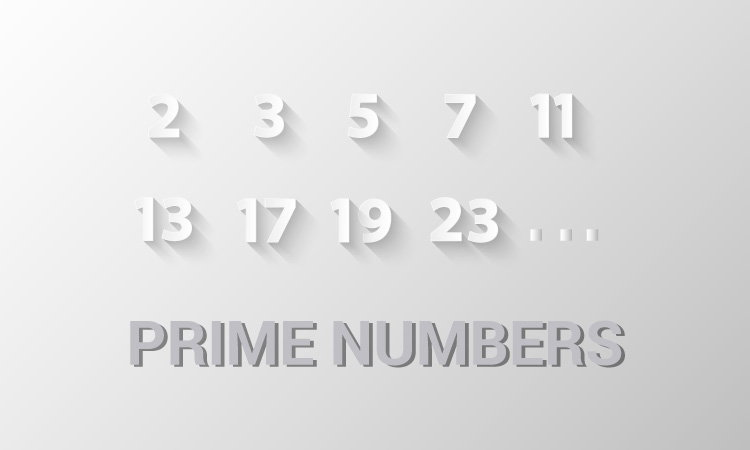# C Program to Display Prime Numbers Between Intervals Using Function

In this example, you will learn to print all prime numbers between two numbers (entered by the user).To understand this example, you should have the knowledge of the following C programming topics:

To find all the prime numbers between the two integers, `checkPrimeNumber()` is created. This function checks whether a number is prime or not.

## Prime Numbers Between Two Integers

``````#include <stdio.h>
int main() {
int n1, n2, i, flag;
printf("Enter two positive integers: ");
scanf("%d %d", &n1, &n2);
printf("Prime numbers between %d and %d are: ", n1, n2);
for (i = n1 + 1; i < n2; ++i) {

// flag will be equal to 1 if i is prime

if (flag == 1)
printf("%d ", i);
}
return 0;
}

// user-defined function to check prime number
int j, flag = 1;
for (j = 2; j <= n / 2; ++j) {
if (n % j == 0) {
flag = 0;
break;
}
}
return flag;
}
``````

Output

```Enter two positive integers: 12
30
Prime numbers between 12 and 30 are: 13 17 19 23 29
```

If the user enters the larger number first, this program will not work as intended. To solve this issue, you need to swap the numbers first.